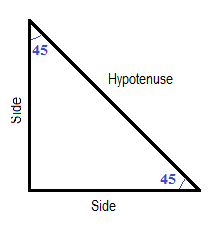## 45 45 Right Triangle Calculator

Note: Input one number then click "calculate" button!

 Side: Hypotenuse: Area: Perimeter:

A 45 45 90 right triangle or right-angled triangle is an Isosceles Triangle. It has two 45 degree angles and one right angle.### 45-45-90 Triangle Formula:

Area = Side × Side / 2.

Perimeter = 2 × Side + √( 2 × Side2)

For example, when side = 1, the hypotenuse = 1.414, area = 0.5, perimeter = 3.414.

For example , when area = 1, the side = 1.414, hypotenuse = 2, perimeter = 4.828427.

Thinkcalculator.com provides you helpful and handy calculator resources.